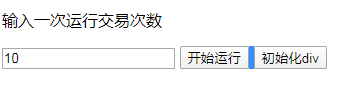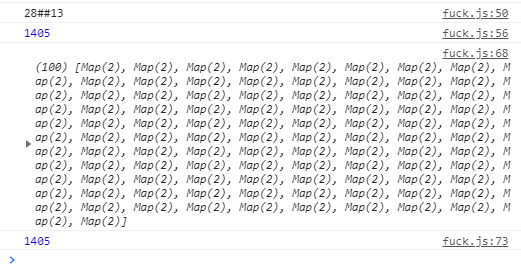function start() {

``````var objects=new Array();
for(var i=1;i<101;i++){
var object=new Map()    ;
object.set('name',i);
object.set('money',10);
objects.push(object)
}
//这里是单纯为验证当前数组里money的总和是多少这里输出是正常的10000
var moneysxm=0;
for(var w=0;w<objects.length;w++){
moneysxm+=objects[w].get('money')
}
console.log(moneysxm);

for(var z=0;z<\$('#f').val();z++){
//随机取两个数
var demo1int=sum(0,99);
var demo2int=sum(0,99);
//获得随机数字对应的数组对象
var demo1object=objects[demo1int];
var demo2object=objects[demo2int];
//获得对象1的金钱数额
var demo1money=demo1object.get('money');
//获得对象2的金钱数额
var demo2money=demo2object.get('money');
console.log(demo1object.get('name')+'##'+demo1money)
console.log(demo2object.get('name')+'##'+demo2money)
//当该对象金钱数额为0时不作为
//如果两个对象是同一个时不作为
if(demo1money!=0||demo1int!=demo2int){
//获得符合该对象当前金钱范围内的随机支出数额
var demo1zhichu=sum(1,demo1money);
//获得对象1的剩余数额
demo1money-=demo1zhichu;
//将对象1剩余数额存入数组
objects[demo1int].set('money',demo1money);
//获得对象二获得数额后的总数额
demo2money+=demo1zhichu;
//将对象2获得数额后的总数额存入数组
objects[demo2int].set('money',demo2money);

}
console.log(objects[demo1int].get('name')+'##'+objects[demo1int].get('money'));
console.log(objects[demo1int].get('name')+'##'+objects[demo2int].get('money'));
}
var moneysxm=0;
for(var s=0;s<objects.length;s++){
moneysxm+=objects[s].get('money')
}
console.log(moneysxm);
//排序
for(var o=0;o<objects.length;o++){
for(var u=0;u<objects.length;u++){
if(objects[o].get('money')<objects[u].get('money')){
var g=objects[o];
objects[o]=objects[u];
objects[u]=g;
}
}
}
setobjects=objects;
console.log(setobjects);
moneysxm=0;
for(var q=0;q<setobjects.length;q++){
moneysxm+=setobjects[q].get('money')
}
console.log(moneysxm);
``````

}

function sum (m,n){
var num = Math.floor(Math.random()*(m - n) + n);
return num;
}

#f是一个输入框java是强类型的，应该不会再出现这种事情了吧。但是显然，我错了。
public class test1234 {

``````public static void main(String[] args) {

ArrayList<persion> arrayList = new ArrayList<persion>();

//创建100个对象
for(int i=1;i<101;i++){
persion persion = new persion();
persion.setName(String.valueOf(i));
persion.setMoney(10);

}

for(int z=0;z<1000;z++){
//随机取两个数
int demo1int=sum(0,99);
int demo2int=sum(0,99);
//获得随机数字对应的数组对象
persion demo1object=arrayList.get(demo1int);
persion demo2object=arrayList.get(demo2int);
//获得对象1的金钱数额
int demo1money=demo1object.getMoney();
//获得对象2的金钱数额
int demo2money=demo2object.getMoney();
//当该对象金钱数额为0时不作为
if(demo1money!=0){
//获得符合该对象当前金钱范围内的随机支出数额
int demo1zhichu=sum(1,demo1money);
//获得对象1的剩余数额
demo1money-=demo1zhichu;
//将对象1剩余数额存入数组
demo1object.setMoney(demo1money);
//获得对象二获得数额后的总数额
demo2money+=demo1zhichu;
//将对象2获得数额后的总数额存入数组
demo2object.setMoney(demo2money);
}
}

int moneysxm=0;
for(int s=0;s<arrayList.size();s++){
moneysxm+=arrayList.get(s).getMoney();
}

System.out.println(moneysxm);

}
java也输出了一些令人害怕的money总量

``````

2个回答

``````            int moneysxm=0;
for(int s=0;s<arrayList.size();s++){
moneysxm+=arrayList.get(s).getMoney();
}
if(demo1money!=0){
System.out.println("本次支出方为" + demo1object.getName() + "收款方为" + demo2object.getName() + "支出为" + demo1zhichu + "当前计算次数" + String.valueOf(z) + "结果为" + moneysxm);
}
``````